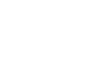Prototype

Rapid Tooling

Contact Us

Tel: +86-13823991970

Email: sales@firstpart.com

Others

More often than not, guess work takes centerstage in mold making and material casting projects. Sadly, most mold experts seldom guess right. This is because estimating the amount of material required to complete a project can be tasking. Consequently, having too little material can disrupt the progress of the project in totality. Having too much can lead to unwanted leftover and waste which is both frustrating and costly.

In order to help with estimations, we take a look at a simple method of estimating material volumes (silicone, gypsum, resin) required to complete a project. Keep in mind that there are many variables to consider including but not limited to the variations in dimensions, undercuts, complexity of the model, complex geometries and drafts. Due to these factors, these figures will not be exact but they will be pretty close. Here’s what to do before starting your next urethane casting project:

## 1. Calculating Resin Coverage Area

There is no fixed formula for estimating both area and material volumes in urethane casting. This is because the area and volume is subject to the shape of the mold to be produced. Often, squares, rectangles, triangles and cylinders are used. An example is given below:

How much epoxy resin is required for a table measuring 5m by 12m?## 2.Calculating the volume of material required

We shall put our focus for rectangular and cylindrical or tube-shaped containers and estimate how to calculate the volume for a project. Irrespective of the shape, to estimate the volume of the material required, you will need to calculate the volume of the casting container and the volume of the shape/pattern to be created. The differences in these two volumes represent the volume of material required.

For Rectangular parts, we shall consider the length, width and height of the container as shown below:The volume of the rectangle is given as Volume (V) = Length * width * height

Let us try to estimate the volume of material needed to make a mold for a 100in3 object using a rectangular mold box that measures 10 by 6 by 4in.

To proceed, we shall first calculate the volume of the mold box:

Volume (v) of mold box = 10” * 6” * 4” = 240in3

Next, we subtract the volume of the object to be made (100m3) from the volume of the mold box:

Volume of material required = volume of mold box – volume of desired part

Volume of material required = 240in3 – 100in3

Volume of material required = 140in3**

** The 140in3 is the volume of the material required to make a 100in3 object. To put this figure in gallon, simply divide this number by 231

Volume of material required in gallon = 140/231 = 0.606 gallons of material.

For cylindrical parts, we shall consider the radius and height of the cylinder used to mold the material. This volume will help to benchmark the possible amount of silicone or resin required to make your own mold. For tube-shaped/cylindrical mold containers, there is a radius and height to be considered.

The volume of material to be used = Volume of mold cylinder – volume of part to be made

Volume of a cylinder is given as V = πr2h. Where r represents the radius of the cylinder and h is the height of the cylinder as in the figure below:Let us consider an example – Calculate the volume of material required to make a cylindrical mold with 25” in height and 10” in diameter, if the mold is to be formed in a cylinder spanning 30” in height with a radius of 8”.

To proceed, we will need to find the radius of the desired cylindrical mold. The radius of any circular shape is half of its diameter. Therefore, in the example above, the radius of the cylindrical mold to be formed is 10”/2, which is 5”.

To calculate the volume of material required (V):

V= volume of cylinder – volume of object to be molded

V = πr2h (cylinder) - πr2h (object to be molded). Where π= 3.142

V = (3.142*(8)2*30) – (3.142*(5)2*25)

V = 6032.64in3 – 1963.75in3

V = 4068.89in3

To get the amount of material required in gallon, divide the volume in cubic inches (in3) by 231 as 1 gallon = 231in3

Volume of material required in gallon = 4068.89/231 = 17.61 gallons

The benefits of being able to accurately estimate the volume of material required for molding operations has numerous benefits. From efficient material management to proper costing and quoting, Firstpart offers excellent injection molding and urethane casting services for all types of molding productions you may require. Our engineers are always available to advice on the best processes, materials estimation and design optimization to ensure cost-savings, quick turnaround and the production of parts that are 100 percent defect and issue free. Click here to request a free quote now and submit your design for a free evaluation and contact us on how to get started on your next projects.

Estimating mold rubber volume for your projects
2021-03-01